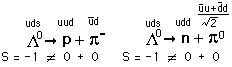# Lambda baryon decays

• I
I've been asked to find the ratio between the cross sections of the two folowing decais:Using the CKM matrix and the feynman diagrams for both decays, I reach the conclusion that the Ratio is exactly 1. However, consulting this document,

http://pdg.lbl.gov/2012/tables/rpp2012-tab-baryons-Lambda.pdf

We clearly see it is not. I do not understand where the problem lies.

My reasoning is the following:
- The decays are exactly the same, except for the final arrangement of the quarks
- Since the vertices are the same, the probability of each interaction is the same in both cases.
- Since the probabilities are the same, the interaction rates are the same.
- Since the mass of the products is roughly equal in both cases, the density of final states is the same
- The cross section is the same.

Staff Emeritus
2021 Award
Two words: Clebsch-Gordon

Two words: Clebsch-Gordon
Are you talking about the fact that the neutral pion is in fact a superposition of two mesons? I thought about that, but I do not understand how I can insert that into Fermi's golden rule and obtain the experimental result.

Ok, after searching for a couple hours I think I found it:

We have a final state with I=1/2 and I3=-1/2. This state is a linear combination of |n, pi0)=|1/2,-1/2)x|1,0) and |p, pi-)=|1/2,1/2)x|1,-1). Therefore, to find the ratio between branches we just need to find a ratio between the coefficients of the linear combination which are the Clebsch-Gordan coefficients.

Is that it?

vanhees71
Gold Member
2021 Award
Two words: Clebsch-Gordon
Clebsch-Gordan

•Staff Emeritus
2021 Award
Got me on that one!

samalkhaiat
Is that it?
Yes, provided you know what all that has to do with the weak decay. Once you “choose” the final state, $|N \pi \rangle$, to be $|1/2 , -1/2\rangle$, you are done: The Clebsch-Gordan expansion gives you
$$| p \pi^{-} \rangle = \sqrt{1/3} \ | \frac{3}{2} , - \frac{1}{2} \rangle - \sqrt{2/3} \ |\frac{1}{2} , - \frac{1}{2} \rangle ,$$
$$|n \pi^{0} \rangle = \sqrt{2/3} \ | \frac{3}{2} , - \frac{1}{2} \rangle + \sqrt{1/3} \ |\frac{1}{2} , - \frac{1}{2} \rangle .$$
Solving these for “the final state”, you get
$$|\frac{1}{2} , -\frac{1}{2} \rangle = -\sqrt{2/3} \ |p \pi^{-} \rangle + \sqrt{1/3} \ |n \pi^{0} \rangle .$$
From this you obtain the result
$$\frac{\sigma \left( \Lambda \to p\pi^{-}\right)}{\sigma \left( \Lambda \to n\pi^{0}\right)} = \frac{(-\sqrt{2/3})^{2}}{(\sqrt{1/3})^{2}} = 2 ,$$ which agrees with the experimental data very well.
Okay, now here are my questions to you:
1) In the final state $|N\pi \rangle$, the nucleon has $I_{N}=\vec{1/2}$ and the pion has $I_{\pi} = \vec{1}$. Therefore, the final state must have $I_{N\pi} = \vec{3/2}$ or $\vec{1/2}$. So, what made you “choose” $|\frac{1}{2} , -\frac{1}{2} \rangle$ to be the final state? What is wrong with the state $|\frac{3}{2} , -\frac{1}{2} \rangle$?
2) Closely related to (1) is the question about the initial state $|\Lambda \rangle$, which we know it is an iso-singlet $|0 , 0\rangle$: In order to find $\sigma (\Lambda \to N\pi)$, we need to evaluate the matrix elements $$T(\Lambda \to N\pi) = \langle N \pi | \mathcal{H}^{|\Delta S| = 1}| \Lambda \rangle ,$$ where $\mathcal{H}^{|\Delta S| = 1}$ is the low energy (non-leptonic) weak Hamiltonian. So, where is the initial state in the above mentioned analysis? Why was there no mention of the initial state $|\Lambda \rangle$?
If you know the answers to those questions, you are okay. Otherwise, you will have to ask better questions.

•vanhees71